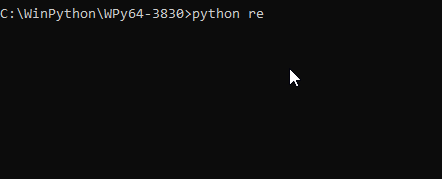# 如何在 Python 中讀取 stdin 中的輸入內容

## 在 Python 中使用 `fileinput.input()` 來讀取 `stdin`

``````Hello
Line1

Line2
``````

``````import fileinput

for line in fileinput.input():
print(line.rstrip())
``````

``````python read.py "sample.txt"
``````

``````Hello
Line1

Line2
``````

``````import fileinput

for line in fileinput.input():
print('Output:', line.rstrip())
``````

``````python read.py
Line 1
Output: Line 1
Line 2
Output: Line2
^Z
````````````import fileinput

data = []
for line in fileinput.input():
data.append(line.rstrip())
``````

## 在 Python 中使用 `sys.stdin` 來讀取 `stdin`

``````import sys
for line in sys.stdin:
print('Output:', line.rstrip())

``````

``````python read.py
Line 1
Output: Line 1
Line 2
Output: Line2
^Z
``````

``````import sys

data = sys.stdin.readlines()
data = [line.rstrip() for line in data]
``````

## 相關文章 - Python Input

• 在 Python 中檢測鍵擊